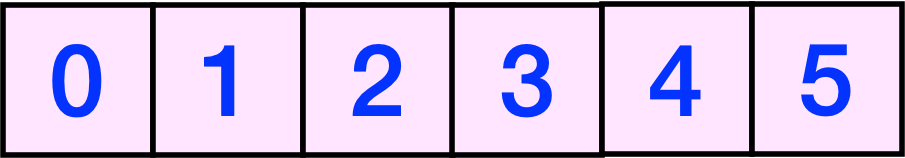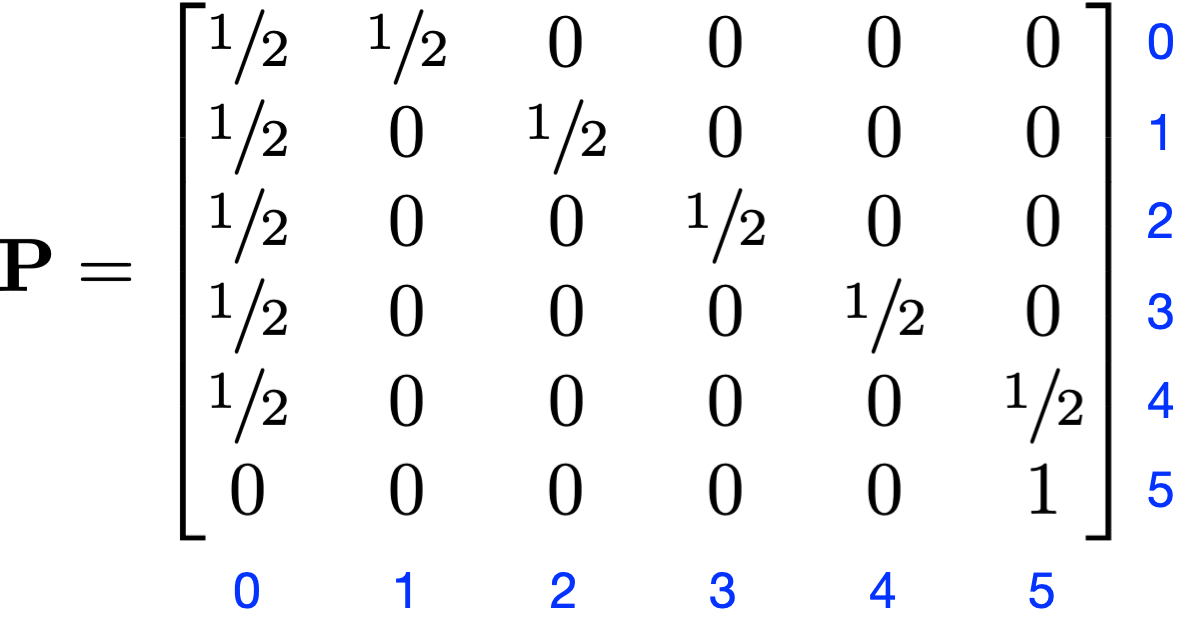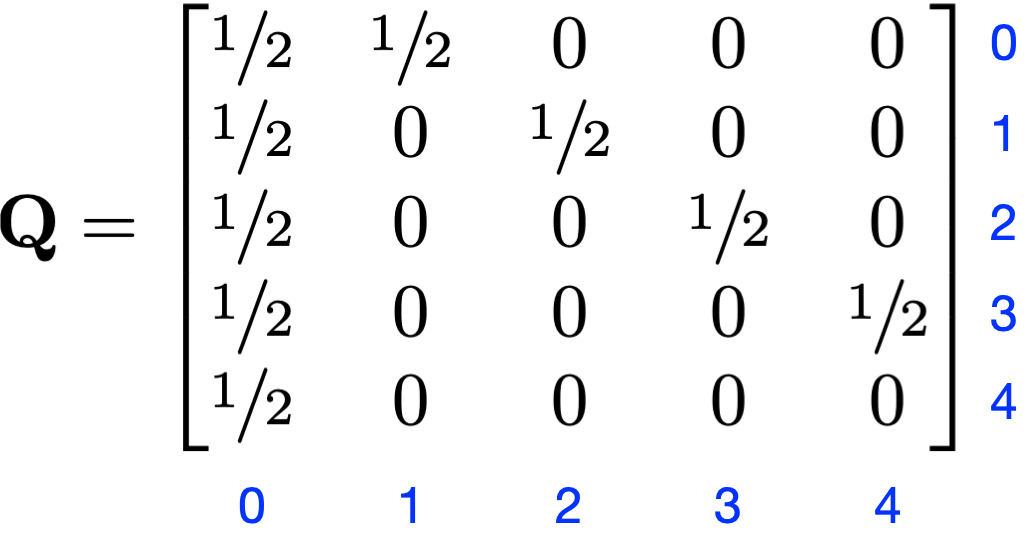In the latest episode of Taskmaster, of which Channel 4 has posted a sneak peek, there’s a subtask related to my last post on Markov chains. The contestants are required to flip a coin and get five consecutive heads before moving on to the next part of the task. As you might expect, there are a few double-headed coins available from earlier in the task, and that makes this subtask very easy for the contestants who notice them. But the subtask can also be done by brute force with a regular coin, which raises the question: how many flips would it take, on average, to accomplish this subtask with a fair coin?

It might be easier to see how Markov chains can be used to answer this question if we build a little board game—an even easier version of Snakes and Ladders—that matches the logic of the coin flip subtask. Imagine a six-square board with the squares labeled from 0 through 5.You start with your marker on the Square 0 and start flipping a coin. If it comes up heads, you move your marker forward; if it comes up tails, you go back to zero. You finish the game when your marker reaches the Square 5, which is the same as flipping 5 heads in a row.

As we did in the earlier post, we’ll take the end square to be an absorbing state and build a transition matrix that reflects the probabilities of moving from a given square to the others. The rows represent the current square and the columns represent the square after the next flip.As you can see, the chance of moving forward one square is always ½, as is the chance of moving back to Square 0. The only square to which this doesn’t apply is Square 5 because that’s where the game ends.

We’ve worked out all the math on this before. We define another transition matrix, $Q$, as the same as $P$ but with the row and column associated with the absorbing state removed:We now define ${m}_{0}$, as the expected number of flips to get from Square 0 to the absorbing state at Square 5. Similar definitions apply to ${m}_{1}$, ${m}_{2}$, ${m}_{3}$, and ${m}_{4}$. Then

$\left(I-Q\right)\phantom{\rule{thinmathspace}{0ex}}m=1$

where $m$ is the column vector of the $m$ terms and $1$ is a five-element column vector of ones.1 Solving this yields

$m=\left[\begin{array}{c}62\\ 60\\ 56\\ 48\\ 32\end{array}\right]$

so the expected number of flips to get five consecutive heads is 62. Certainly doable but also frustrating—a common Taskmaster state of affairs.

You might be looking at the 32 at the end of $m$ and thinking it’s too high to be the expected number of flips needed to get from four consecutive heads to the fifth consecutive head. While it’s true that you could get from Square 4 to Square 5 in one flip, the problem is that it’s equally likely that that flip will take you back to Square 0. So the expected number of flips to get from Square 4 to Square 5 is

$\left(1\right)\phantom{\rule{thinmathspace}{0ex}}\frac{1}{2}+\left(62+1\right)\phantom{\rule{thinmathspace}{0ex}}\frac{1}{2}=32$

just as we calculated via the matrix equation.

1. I’m using bold for the vectors and matrices because that’s the common typographical convention. I didn’t use bold in the previous post because Marcus du Sautoy didn’t, and I wanted to be consistent with him.